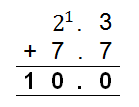Mathematics
Easy

Question

# Sum of 2.3 + 7.7

## 9101112Hint:

## The correct answer is: 10

### Subtract 0.3 from 2.3 and compensate by adding 0.3 to 7.7 (2.3 - 0.3) + (7.7 + 0.3) 2 + 8 = 10

Arrange the numbers vertically and add:Step 1: Add 3 and 7.

3+7=10

Here, the 1 is a carry.

Step 2:

2 + 7 + 1 = 10

The correct option is (b) 10

### Related Questions to study#### With Turito Foundation.#### Get an Expert Advice From Turito.